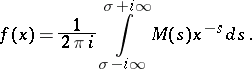# Mellin transform

The integral transform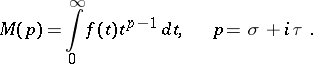The substitutionreduces it to the Laplace transform. The Mellin transform is used for solving a specific class of planar problems for harmonic functions in a sectorial domain, of problems in elasticity theory, etc.

The inversion theorem. Suppose that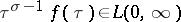and that the functionhas bounded variation in a neighbourhood of the point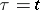. ThenThe representation theorem. Suppose that the functionis summable with respect toon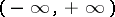and has bounded variation in a neighbourhood of the point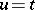. Then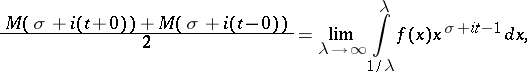where